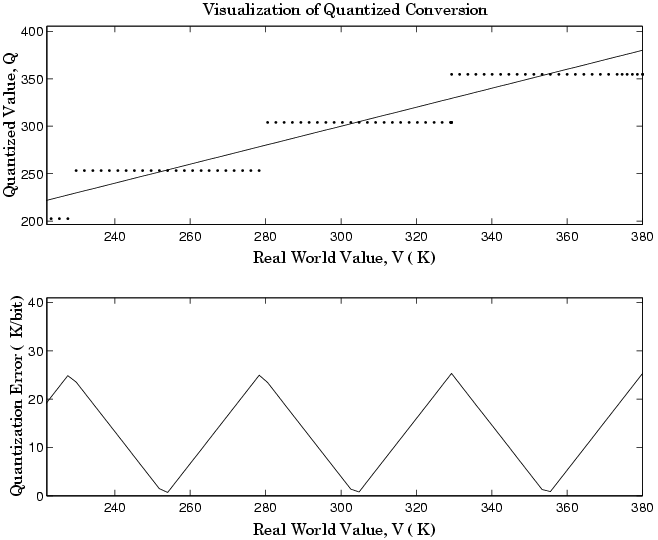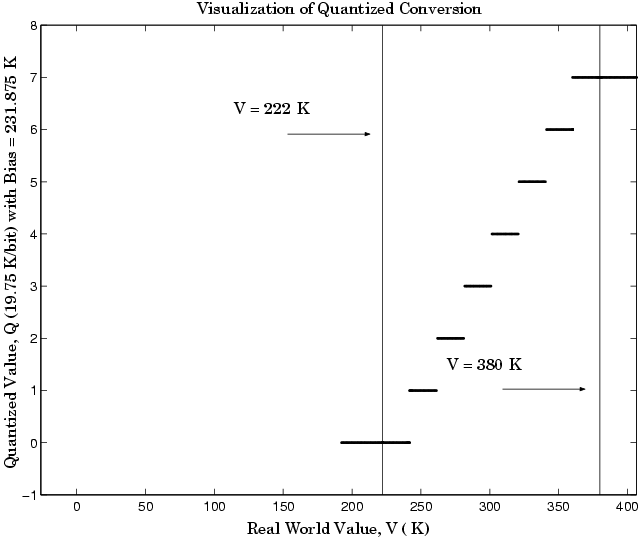Physical Quantities and Measurement Scales

Introduction

The decision to use fixed-point hardware is simply a choice to represent numbers in a particular form. This representation often offers advantages in terms of the power consumption, size, memory usage, speed, and cost of the final product.

A measurement of a physical quantity can take many numerical forms. For example, the boiling point of water is 100 degrees Celsius, 212 degrees Fahrenheit, 373 kelvin, or 671.4 degrees Rankine. No matter what number is given, the physical quantity is exactly the same. The numbers are different because four different scales are used.

Well known standard scales like Celsius are convenient for the exchange of information. However, there are situations where it makes sense to create and use unique nonstandard scales. These situations usually involve making the most of a limited resource.

For example, nonstandard scales allow map makers to get the maximum detail on a fixed size sheet of paper. A typical road atlas of the USA will show each state on a two-page display. The scale of inches to miles will be unique for most states. By using a large ratio of miles to inches, all of Texas can fit on two pages. Using the same scale for Rhode Island would make poor use of the page. Using a much smaller ratio of miles to inches would allow Rhode Island to be shown with the maximum possible detail.

Fitting measurements of a variable inside an embedded processor is similar to fitting a state map on a piece of paper. The map scale should allow all the boundaries of the state to fit on the page. Similarly, the binary scale for a measurement should allow the maximum and minimum possible values to fit. The map scale should also make the most of the paper in order to get maximum detail. Similarly, the binary scale for a measurement should make the most of the processor in order to get maximum precision.

Use of standard scales for measurements has definite compatibility advantages. However, there are times when it is worthwhile to break convention and use a unique nonstandard scale. There are also occasions when a mix of uniqueness and compatibility makes sense. See the sections that follow for more information.

Selecting a Measurement Scale

Suppose that you want to make measurements of the temperature of liquid water, and that you want to represent these measurements using 8-bit unsigned integers. Fortunately, the temperature range of liquid water is limited. No matter what scale you use, liquid water can only go from the freezing point to the boiling point. Therefore, this is the range of temperatures that you must capture using just the 256 possible 8-bit values: 0,1,2,...,255.

One approach to representing the temperatures is to use a standard scale. For example, the units for the integers could be Celsius. Hence, the integers 0 and 100 represent water at the freezing point and at the boiling point, respectively. On the upside, this scale gives a trivial conversion from the integers to degrees Celsius. On the downside, the numbers 101-255 are unused. By using this standard scale, more than 60% of the number range has been wasted.

A second approach is to use a nonstandard scale. In this scale, the integers 0 and 255 represent water at the freezing point and at the boiling point, respectively. On the upside, this scale gives maximum precision since there are 254 values between freezing and boiling instead of just 99. On the downside, the units are roughly 0.3921568 degrees Celsius per bit so the conversion to Celsius requires division by 2.55, which is a relatively expensive operation on most fixed-point processors.

A third approach is to use a “semistandard” scale. For example, the integers 0 and 200 could represent water at the freezing point and at the boiling point, respectively. The units for this scale are 0.5 degrees Celsius per bit. On the downside, this scale does not use the numbers from 201-255, which represents a waste of more than 21%. On the upside, this scale permits relatively easy conversion to a standard scale. The conversion to Celsius involves division by 2, which is an easy shift operation on most processors.

Measurement Scales: Beyond Multiplication

One of the key operations in converting from one scale to another is multiplication. The preceding case study gave three examples of conversions from a quantized integer value Q to a real-world Celsius value V that involved only multiplication:

Graphically, the conversion is a line with slope S, which must pass through the origin. A line through the origin is called a purely linear conversion. Restricting yourself to a purely linear conversion can be wasteful and it is often better to use the general equation of a line:

V = SQ + B.

By adding a bias term B, you can obtain greater precision when quantizing to a limited number of bits.

The general equation of a line gives a useful conversion to a quantized scale. However, like all quantization methods, the precision is limited and errors can be introduced by the conversion. The general equation of a line with quantization error is given by

$V=SQ+B±Error.$

If the quantized value Q is rounded to the nearest representable number, then

$-\frac{S}{2}\le Error\le \frac{S}{2}.$

That is, the amount of quantization error is determined by both the number of bits and by the scale. This scenario represents the best-case error. For other rounding schemes, the error can be twice as large.

Select a Measurement Scale for Temperature

On typical electronically controlled internal combustion engines, the flow of fuel is regulated to obtain the desired ratio of air to fuel in the cylinders just prior to combustion. Therefore, knowledge of the current air flow rate is required. Some manufacturers use sensors that directly measure air flow, while other manufacturers calculate air flow from measurements of related signals. The relationship of these variables is derived from the ideal gas equation. The ideal gas equation involves division by air temperature. For proper results, an absolute temperature scale such as kelvin or Rankine must be used in the equation. However, quantization directly to an absolute temperature scale would cause needlessly large quantization errors.

The temperature of the air flowing into the engine has a limited range. On a typical engine, the radiator is designed to keep the block below the boiling point of the cooling fluid. Assume a maximum of 225oF (380 K). As the air flows through the intake manifold, it can be heated to this maximum temperature. For a cold start in an extreme climate, the temperature can be as low as -60oF (222 K). Therefore, using the absolute kelvin scale, the range of interest is 222-380 K.

The air temperature needs to be quantized for processing by the embedded control system. Assuming an unrealistic quantization to 3-bit unsigned numbers: 0,1,2,...,7, the purely linear conversion with maximum precision is

The quantized conversion and range of interest are shown in the following figure.Notice that there are 7.5 possible quantization values. This is because only half of the first bit corresponds to temperatures (real-world values) greater than zero.

The quantization error is –25.33 K/bit ≤ Error ≤ 25.33 K/bit.

The range of interest of the quantized conversion and the absolute value of the quantized error are shown in the following figure.As an alternative to the purely linear conversion, consider the general linear conversion with maximum precision:

The quantized conversion and range of interest are shown in the following figure.The quantization error is -9.875 K/bit ≤ Error ≤ 9.875 K/bit, which is approximately 2.5 times smaller than the error associated with the purely linear conversion.

The range of interest of the quantized conversion and the absolute value of the quantized error are shown in the following figure.Clearly, the general linear scale gives much better precision than the purely linear scale over the range of interest.

Fixed-Point Designer DocumentationGet trial now